Courses

# Thernodynamic Properties Physics Notes | EduRev

## Physics : Thernodynamic Properties Physics Notes | EduRev

The document Thernodynamic Properties Physics Notes | EduRev is a part of the Physics Course Basic Physics for IIT JAM.
All you need of Physics at this link: Physics

Equations of State:
The equation of state for a substance provides the additional information required to calculate the amount of work that the substance does in making a transition from one equilibrium state to another along some specified path. The equation of state is expressed as a functional relationship connecting the various parameters needed to specify the state of the system. The basic concepts apply to all thermodynamic systems, but here, in order to make the discussion specific, a simple gas inside a cylinder with a movable piston will be considered. The equation of state then takes the form of an equation relating P, V, and T, such that if any two are specified, the third is determined. In the limit of low pressures and high temperatures, where the molecules of the gas move almost independently of one another, all gases obey an equation of state known as the ideal gas law: PV = nRT, where n is the number of moles of the gas and R is the universal gas constant, 8.3145 joules per K. In the International System of Units, energy is measured in joules, volume in cubic metres (m3), force in newtons (N), and pressure in pascals (Pa), where 1 Pa = 1 N/m2. A force of one newton moving through a distance of one metre does one joule of work. Thus, both the products PV and RT have the dimensions of work (energy). A P-V diagram would show the equation of state in graphical form for several different temperatures.
To illustrate the path-dependence of the work done, consider three processes connecting the same initial and final states. The temperature is the same for both states, but, in going from state i to state f, the gas expands from Vi to Vf (doing work), and the pressure falls from Pi to Pf. According to the definition of the integral in equation (22), the work done is the area under the curve (or straight line) for each of the three processes. For processes I and III the areas are rectangles, and so the work done is
WI = Pi(Vf − Vi)      (23)
and
WIII = Pf(Vf − Vi),   (24)

respectively. Process II is more complicated because P changes continuously as V changes. However, T remains constant, and so one can use the equation of state to substitute P = nRT/V in equation (22) to obtain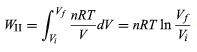(25)

or, because
PiVi = nRT = PfVf       (26)
for an (ideal gas) isothermal process,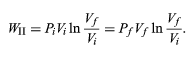(27)

WII is thus the work done in the reversible isothermal expansion of an ideal gas. The amount of work is clearly different in each of the three cases. For a cyclic process the net work done equals the area enclosed by the complete cycle.

The Clausius-Clapeyron equation:
Phase changes such as the conversion of liquid water to steam, provide an important example of a system in which there is a large change in internal energy with volume at constant temperature. Suppose that the cylinder contains both water and steam in equilibrium with each other at pressure P, and the cylinder is held at constant temperature T, as shown in the figure. The pressure remains equal to the vapour pressure Pvap as the piston moves up, as long as both phases remain present. All that happens is that more water turns to steam, and the heat reservoir must supply the latent heat of vaporization, λ = 40.65 kilo joules per mole, in order to keep the temperature constant.
The results of the preceding section can be applied now to find the variation of the boiling point of water with pressure. Suppose that as the piston moves up, 1 mole of water turns to steam. The change in volume inside the cylinder is then ΔV = VgasVliquid, where Vgas = 30.143 litres is the volume of 1 mole of steam at 100 °C, and Vliquid = 0.0188 litre is the volume of 1 mole of water. By the first law of thermodynamics, the change in internal energy ΔU for the finite process at constant P and T is ΔU = λ − PΔV.

The variation of U with volume at constant T for the complete system of water plus steam is thus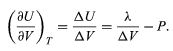(48)

A comparison with equation then yields the equation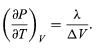(49)

However, for the present problem, P is the vapour pressure Pvapour, which depends only on T and is independent of V. The partial derivative is then identical to the total derivative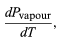giving the Clausius-Clapeyron equation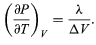(51)

This equation is very useful because it gives the variation with temperature of the pressure at which water and steam are in equilibrium—i.e., the boiling temperature. An approximate but even more useful version of it can be obtained by neglecting Vliquid in comparison with Vgas and using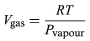(52)

from the ideal gas law. The resulting differential equation can be integrated to give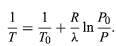(53)

For example, at the top of Mount Everestatmospheric pressure is about 30 percent of its value at sea level. Using the values R = 8.3145 joules per K and λ = 40.65 kilojoules per mole, the above equation gives T = 342 K (69 °C) for the boiling temperature of water, which is barely enough to make tea.

Offer running on EduRev: Apply code STAYHOME200 to get INR 200 off on our premium plan EduRev Infinity!

## Basic Physics for IIT JAM

109 videos|157 docs|94 tests

,

,

,

,

,

,

,

,

,

,

,

,

,

,

,

,

,

,

,

,

,

;